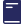Over 1000 Titles for students & teachers

# Excel Study Guide - Mathematics Year 7

\$34.95

This Excel Mathematics Study Guide is essential for all students studying Year 7 Mathematics as a comprehensive guide to the topics covered at this level. It provides tools to explain important mathematical concepts and gives ample opportunity to practise at a variety of levels.

Excel Mathematics Study Guide Year 7 contains:

• Topics covered in Year 7 Mathematics courses in Australia
• Explanations of important concepts with examples and worked solutions
• Checklists at the end of each chapter with page references to explanations
• Plenty of exercises in the \ Practise sections at the end of each chapter; worked solutions and a marking system that provides feedback are provided
• Three levels of timed tests (straightforward, average difficulty and challenging) at the end of each chapter; worked solutions and a marking system that provides feedback are provided
• Three levels of timed Sample Exam Papers (straightforward, average difficulty and challenging) that cover the entire year's work and provide preparation for the final Year 7 examination; worked solutions are provided
• A comprehensive index
CHAPTERS:

1. Number
2. Fractions
3. Decimals
4. Percentages
5. Integers
6. Ratios
7. Algebra
8. Measurement
9. Geometry
10. Equations
11. Statistics and Probability
12. Sample Examinations
14. Index

#### Product Details

SKU:
9781741250060
YEAR:
Year 7
Publication Edition Date:
2014/01
Dimensions:
280 mm (h) x 210 mm (w)
ISBN:
9781741250060
Author(s):
Allyn Jones
BRAND:
Excel
SUB BRAND:
Excel Study Guides
Author(s):
John Compton
SUBJECT:
Mathematics
Format:
Paperback
Author(s):
Peter Nicolas
TYPE:
Study Guides